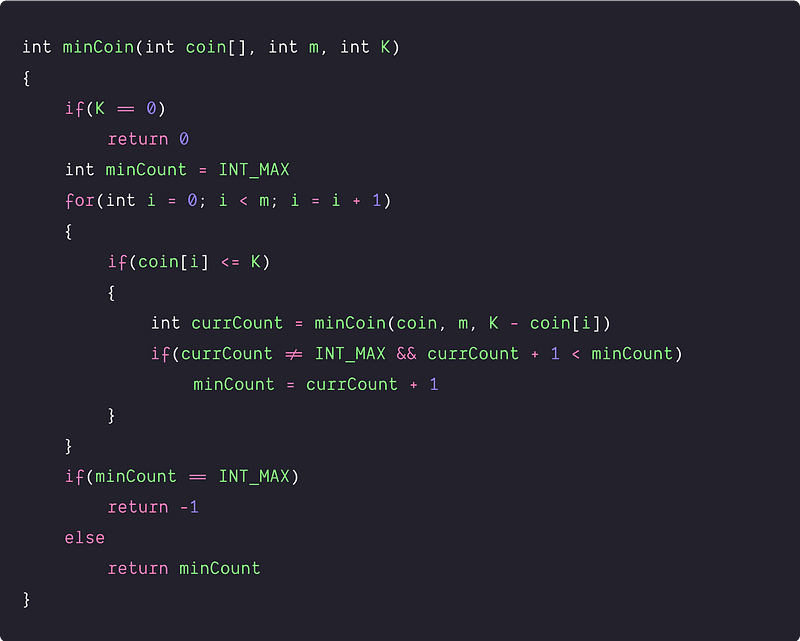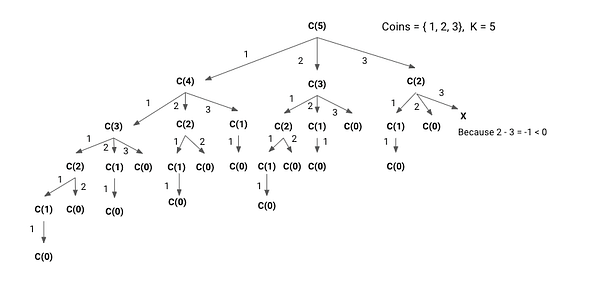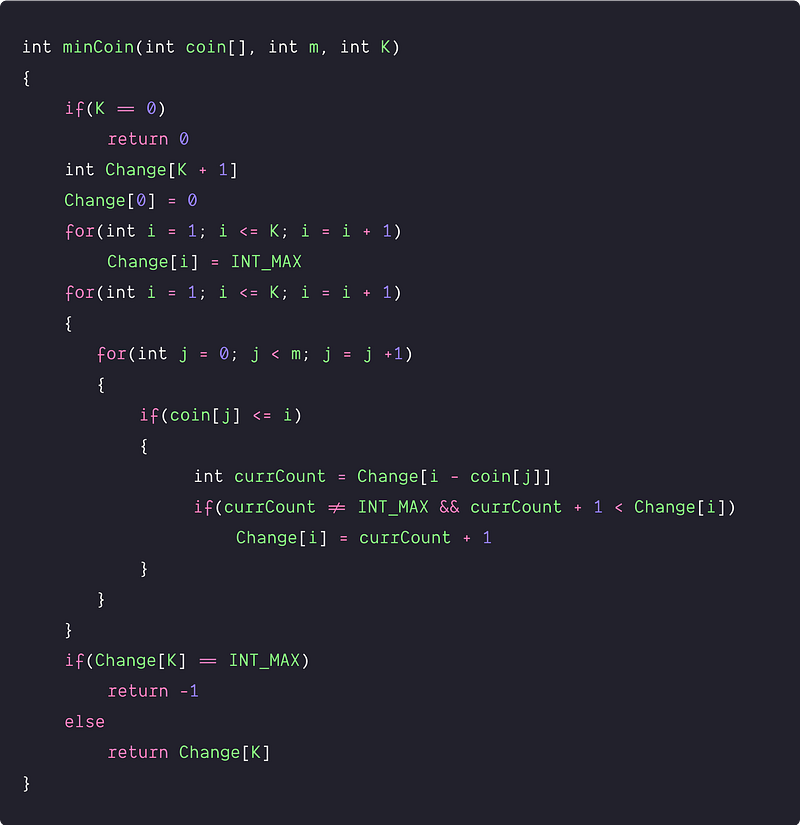# Minimum Coin Change

Difficulty: Medium, Asked-in: Microsoft, Amazon, Morgan Stanley

Key takeaway: An excellent optimization problem to learn problem-solving using dynamic programming.

### Let’s understand the problem

We are given an amount K representing a total amount of money and an integer array coin[] of size m representing coins of different denominations. Write a program to find the minimum number of coins required to make the change.

• We may assume that we have an infinite supply of each kind of coin with the value coin to coin[m-1]. If any combination of the coins cannot make up amount K of money, return -1.

Example 1

Input: coin[] = [25, 10, 5], K = 30, Output: 2

Explanation: Minimum 2 coins required: we can use one coin of 25 and one coin of 5.

Example 2

Input: coin[] = [9, 6, 5, 1], K = 13, Output: 3

Explanation: Minimum 3 coins required: we can use two coins of 6 and one coin of 1.

Example 3

Input: coin[] = [1, 3, 5, 7], K = 18, Output: 4

Explanation: Minimum 4 coins required. We can use any one of these combinations to provide change using 4 coins: (7, 7, 3, 1),(5, 5, 5, 3), and (7, 5, 5, 1).

### Discussed solution approaches

• A brute force approach  using recursion
• An efficient approach  using the bottom-up approach of DP

### A brute force approach  using recursion

Solution Idea and Steps

This is an optimization problem because there can be several ways to provide change, but we need to return the change using the minimum number of coins. In different words :  solution space is huge and only a few solutions will provide the optimal solution. So one basic idea would be to explore all solutions or possibilities of the change and return the count of the solution with a minimum number of coins. But the critical question is :  how do we implement this? Let’s think!

• In such a situation, when we need to explore all possibilities, we can think about solving the problem recursively, i.e. the solution of the problem using smaller sub-problems.
• Initially available choices are important for building the recursive solution. Here we have m choices of the coin in the start i.e. we can pick any coin among m coins.
• Suppose minCoin(coin[], m, K) returns the minimum number of coins required to make a change of value K (This is our larger problem). If we select any coin[i] first, then the smaller sub-problem is minCoin(coin[], m, K  - coin[i]) i.e. the minimum number of coins required to make a change of amount K -  coin[i].
• So for i = 0 to m-1, whichever choice provides the change using the minimum number of coins, we shall add 1 and return the value. But before selecting any coin, make sure whether the value of the coin is less than equal to the amount needed i.e. coin[i] <= K.
• Recursive structure:

minCoin(coin[], m, K) = min (for i = 0 to m-1) { 1 + minCoin(coin[], m, K - coin[i]) }

Where coin[i] <= K

• Base case: If K == 0, then 0 coins required.

Solution PseudocodeTime and space complexity analysis

From the above observation, if we increase the value of K or the value of m, then the total number of recursive calls will increase. So the time complexity depends on both K and m i.e. Input size = (K, m).

For the upper bound of the worst-case analysis, suppose coin[i] <= K during the recursion and, the recursion tree would be a full tree where each child has m nodes. So we have m number of recursive calls at the 1st level of the recursion tree, m^2 number of recursive calls at the 2nd level of the recursion tree, and so on.

Total number of recursive calls in the worst-case = 1 + m + m^2 + ... m^k = (m^k - 1) / (m -1) = O(m^k), which is an exponential time. Note: Sum of the geometric progression S = a + ar + ar^2 +...+ ar^n = a(r^n - 1)/(r - 1), where r > 1. In retrospect, this is not surprising because we are exploring all the possibilities to provide the change. If we create a recursion tree for the above approach and clearly notice the overlapping or repeated subproblems.

For example, the following picture is the recursion tree diagram for K = 5 and coins of the given values [1, 2, 3]. The Sub-problem of size 3 is coming 2 times, the sub-problem of size 2 is coming 4 times, sub-problem of size 1 is coming 7 times.### An efficient approach  using the bottom-up approach of DP

Solution Idea and Steps

Since we have identified that it is a dynamic programming problem, we can solve it using the bottom-up approach. Our aim here is to calculate the solution of the smaller problems in an iterative way and store their result in a table. But the critical question is :  how do we build the solution in a bottom-up manner? Let’s think!

• Table structure: The state of the smaller sub-problems depends on the one variable K because it decreases after each recursive call. So we need to construct a 1-D table to store the solution of the sub-problems.
• Table size: The size of the 1-D table is equal to the total different subproblems. According to the recursion tree, there can be a total (K + 1) of different sub-problems i.e sub-problems of size (K, K-1,…2, 1, 0). int Change[K + 1]
• Table initialization: Before building the solution using the iterative structure of the bottom-up approach, we need to initialize the table by the smaller version of the solution i.e base case. Change = 0
• Iterative structure to fill the table: Now, we need to define the iterative structure to fill the table Change[i] i.e a relation by which we build the solution of the larger problem using the solution of smaller problems in a bottom-up manner. We can easily define the iterative structure by using the recursive structure of the recursive solution.

Change[i] = min (for j = 0 to m-1) { 1 + Change[i - coin[j]] }, where coin[j] <= K

5. Returning final solution: After filling the table iteratively, our final solution gets stored at the last Index of the array i.e.return Change[K].

Solution PseudocodeTime and space complexity analysis

There are two nested loops in the code. The first loop is iterating from 1 to K and the second is iterating from 0 to m-1. Time Complexity = O(K*m)

Space Complexity = O(K), for extra array Change[] of size K + 1.

### Critical ideas to think!

• Do we require the entire array of size equal to K or can we optimize the space? Think about the case when the amount is too high.
• How can we modify the code to find the coins which are part of the optimal solution?
• Can we solve this problem using some other approach? Can we think of applying the greedy or divide and conquer strategy?
• Explore DP problems that can be solved using a similar idea.
• Why is the recursive solution exponential time? Try to analyze the recursive code using the recursion tree method.
• Think about the top-down approach to solve this problem

### Suggested problems to solve

• Find the number of ways in which you can change an amount with given coins of different denominations.
• Greedy algorithm to find minimum coin count.
• Rod cutting problem
• 0–1 Knapsack problem
• Weighted job scheduling

Enjoy learning, Enjoy algorithms!

Get well-designed application and interview centirc content on ds-algorithms, machine learning, system design and oops. Content will be delivered weekly.